Double Integrals In Polar Coordinates

(requires JavaScript)

1. For each region shown, decide whether to use polar or rectangular coordinates and write the iterated integral $\underset{R}{\iint }f\left(x,y\right)\phantom{\rule{0.2em}{0ex}}dA$, where $f$ is an arbitrary continuous function.

a.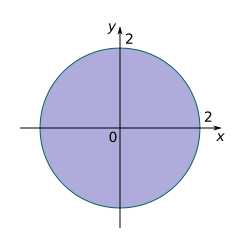b.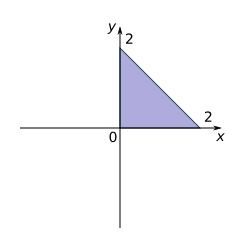c.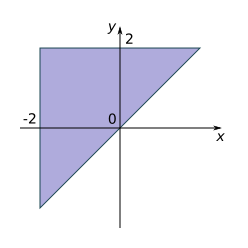d.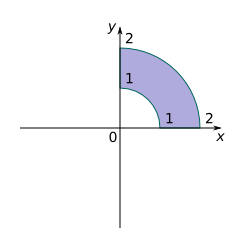e.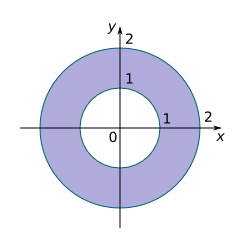f.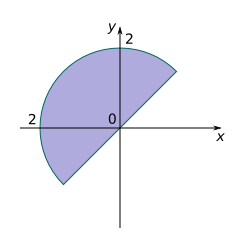2. Sketch the region whose area is given by the integral and evaluate the integral

${\int }_{0}^{\pi /2}{\int }_{0}^{4\mathrm{cos}\left(\theta \right)}r\phantom{\rule{0.2em}{0ex}}dr\phantom{\rule{0.2em}{0ex}}d\theta$

3. Evaluate the integral

$\underset{R}{\iint }\mathrm{cos}\left({x}^{2}+{y}^{2}\right)\phantom{\rule{0.2em}{0ex}}dA$

by changing to polar coordinates. Here $R$ is the region that lies above the $x\text{-axis}$ within the circle ${x}^{2}+{y}^{2}=9$.

$\frac{1}{2}\pi \mathrm{sin}\left(9\right)$
4. Evaluate the integral

$\underset{R}{\iint }{\mathrm{tan}}^{-1}\left(y/x\right)\phantom{\rule{0.2em}{0ex}}dA$

by changing to polar coordinates, given that

$R=\left\{\left(x,y\right)|1\le {x}^{2}+{y}^{2}\le 4,\phantom{\rule{0.5em}{0ex}}0\le y\le x\right\}$

$\frac{3}{64}{\pi }^{2}$
5. Use polar coordinates to find the volume of the solid above the cone $z=\sqrt{{x}^{2}+{y}^{2}}$ and below the sphere ${x}^{2}+{y}^{2}+{z}^{2}=1$.
$\frac{2\pi }{3}\left(1-\frac{1}{\sqrt{2}}\right)$
6. Use polar coordinates to find the volume of the solid inside both the cylinder ${x}^{2}+{y}^{2}=4$ and the ellipsoid $4{x}^{2}+4{y}^{2}+{z}^{2}=64$.
$\frac{8\pi }{3}\left(64-24\sqrt{3}\right)$
7. Evaluate the iterated integral ${\int }_{-3}^{3}{\int }_{0}^{\sqrt{9-{x}^{2}}}\mathrm{sin}\left({x}^{2}+{y}^{2}\right)\phantom{\rule{0.2em}{0ex}}dy\phantom{\rule{0.2em}{0ex}}dx$ by converting to polar coordinates.
$\frac{1}{2}\pi \left(1-\mathrm{cos}\left(9\right)\right)$
8. Evaluate the iterated integral ${\int }_{0}^{2}{\int }_{0}^{\sqrt{2x-{x}^{2}}}\sqrt{{x}^{2}+{y}^{2}}\phantom{\rule{0.2em}{0ex}}dy\phantom{\rule{0.2em}{0ex}}dx$ by converting to polar coordinates.
$\frac{16}{9}$